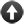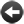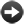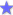§15.8. Units

Suppose we want to talk about how tall people are. We could just create a "number" property, like this:

A person has a number called height.

But then we would have to write lines like "Isabella has height 68", which nobody would naturally say. What we want is to be able to write "Isabella is 5 foot 8." Perhaps the computer will need to store that measurement as the number 68 in some register or other, but we don't want to know about that.

"5 foot 8" is a complicated notation in a way - it involves both feet and inches - so let's start with a simpler example:

A weight is a kind of value. 10kg specifies a weight.

This is a little different to the kinds of value seen so far, which were all created like so:

A colour is a kind of value. The colours are red, green and blue.

We can't mix the two styles: a new kind of value will either be numerical at heart ("10kg") or verbal at heart ("blue").

The effect of "10kg specifies a weight" is to tell Inform that this is the notation for writing a constant "weight". So, for instance,

The maximum load is a weight that varies. The maximum load is 8000kg.

if the maximum load is greater than 8000kg, ...

Inform is then careful not to allow weights to be mixed up with other numerical values. For instance, it won't allow "if the maximum load is 400", because 400 is a number, not a weight.

More or less anything we can do with numbers, we can now do with weights. For instance, we can write:

The Weighbridge is a room. "A sign declares that the maximum load is [maximum load]."

...which will produce the text "A sign declares that the maximum load is 8000kg."

Numerical kinds of value are sometimes called "units", because one of their main uses is to allow us to write quantities using scientific units such as kilograms. But they have other uses too. We have a great deal of freedom in creating notations like "10kg", or "4 foot 10" - the main thing is that new notations must not already mean a value. So "10 specifies a weight" will not be allowed, because 10 specifies a number already.

By default we can only write whole-number values. As we've seen, Inform can handle both integer (whole-number) and real arithmetic, and they each have their advantages. The default here is to use whole numbers, so

10 kg specifies a weight.

will store only whole numbers of kilograms (unless clever scaling tricks are used: see the next section). That may be fine, but if we need to handle a wider range of weights, or do scientific calculations that need to be more accurate, this is better:

1.0 kg specifies a weight.

Here Inform can see from the ".0" in the prototype number that real numbers will be involved. (It needs to be ".0" not, say, ".5" because that could be read as a different sort of notation.) We can still write "8000kg", but we can now also write "1.9885 x 10^30 kg" (the mass of the Sun) or "9.109383 x 10^−31 kg" (the mass of an electron). On the other hand, any calculations we do will be limited in accuracy to about 6 to 9 decimal places, exactly as for real numbers.

By default we can only write positive values when whole numbers are used. Sometimes it is unnatural to write negative values, and so Inform will issue a Problem message if this is tried - for instance, Inform would not allow us to write a weight of -4 kg. (This doesn't mean that arithmetic on units is forbidden to get a negative result: we may want to work out the difference between two weights. Inform's Problem message is simply to try to prevent the accidental writing of incorrect values.) If we do want the ability to write negative values in the source text, we signal that in the notation itself:

-10 kg specifies a weight.

That alerts Inform that both positive and negative values for this unit make sense.

If we set up a spread of multiple notations (see the next section) then this is automatically enabled, because then we're clearly dealing with proper physics, where negative values are common; and similarly if we use real numbers (as above).Start of Chapter 15: Numbers and EquationsBack to §15.7. TrigonometryOnward to §15.9. Multiple notationsExamplerBGH The player character's height is selected randomly at the start of play.ExampleLethal Concentration 1 A poisonous gas that spreads from room to room, incapacitating or killing the player when it reaches sufficient levels.ExampleWonderland Hiking Mount Rainier, with attention to which locations are higher and which lower than the present location.